## Problem statement

Each new term in the Fibonacci sequence is generated by adding the previous twi terms. By starting with 1 and 2, the first 10 terms will be: 1, 2, 3, 5, 8, 13, 21, 34, 55, 89, ... By considering the terms in the Fibonacci sequence whose values do not exceed four million, find the sum of the even valued terms.

## Thoughts

This can obviously be solved with a loop, and indeed every solution I have seen online solves it that way. My gut instinct tells me it can be solved without loops though.

## Solution

### Sum of fibonacci numbers

Can we find a formula for the sum of fibonacci numbers? There is a fairly well known solution for this, so I won't explain it all again. # You can look it up if you are unfamiliar, but it boils down to this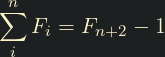### Sum of even fibonacci numbers

Okay, so we can sum fibonacci numbers, but can we sum even fibonacci numbers? # This took me a little longer to clock, but it turns out that yes we can! Lets look at a few numbers at the beginning of the sequence

nFnSumEven sum
1110
2120
3242
4372
55122
682010
7133310
8215410
9348844
105514344
118923244
12144376188
13233609188
14377986188

The first thing we can notice is that every third fibonacci number is even. But what we can also see is that, for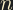being even, the sum of the even fibonacci numbers up tois equal to half the sum of all fibonacci numbers up toLook, for example, the 6th fibonacci number, 8. If we sum all the even fibonacci numbers up to 8 we get 10, which is half the 20 value in the sum column

So it looks like, forbeing even, we can sum all the even values up towith the following: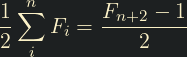### Finding the value at n

We can find the sum of all fibonacci numbers up toif we know the value of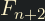. There is also a well-known solution to this problem, using Binet's formula: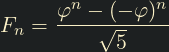### Finding n

The above formulae are only useful if we know. Currently, we don't. We just know that we wantto be the index of whatever the largest fibonacci number under four million is. How do we find that?

Thanks to [a helpful answer I found on Quora] (https://www.quora.com/Given-a-number-N-how-do-I-efficiently-find-the-closest-Fibonacci-number-N) from user Amitabha Tripathi, I found that we can rearrange Binet's formula to find the index of the largest number under a given limit.

For upper bound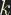the index of the largest fibonacci number belowis given by: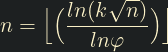## Putting it all together

• Find the indexof the largest fibonacci number below the target
• Findfor the largest even fibonacci number below this value n = n - n % 3
• Use Binet's formula to get the value of• Subtract 1 to find the sum of all fibonacci numbers up to• Divide by 2 to find the sum of all even fibonacci numbers up to this point
#include <math.h>
const double gr = (1 + sqrt(5)) / 2; /* Golden ratio */
const double grRecip = 1 / gr; /* Golden ratio reciprocal */

double binet(int n)
{
return (pow(gr, n) - pow((-grRecip), n)) / sqrt(5);
}

int GetSumEvenUnder(int limit)
{
/* Calculate $n$ for the largest even number below the limit */
int n = floor(log(limit * sqrt(5)) / log(gr));
n = n - n % 3;

float sumToN = binet(n + 2);

return sumToN / 2;
}

#include <stdio.h>
int main()
{
printf("%d\n", GetSumEvenUnder(4000000));
}